# Python Matplotlib for Machine Learning.

## About Matplolib Library in Python

Machine Learning is all about playing with data in order to give your machine more insight about what it can learn, Python is well known and favorite programming language among machine learning developers because of its vast libraries that helps in data exploration. In today’s blog, we are going to talk about the matplotlib library that one should know in detail. So here we go,

The three most important libraries that every Machine Learning developer is going to use in most of the basic, as well as advanced machine learning algorithms, are:-

1. Matplotlib
2. Numpy
3. Pandas

We will know about the purpose of the matplotlib library and its practical implementation, let’s start

Matplotlib  was scripted by  John D.Hunter(it’s always good to know about the creator),  Michael Droettboom  and  Thomas Caswell  joined the matplotlib development later. This library is generally used in association with numpy(we will discuss later), Matplotlib plots the data for visualization for more data insight.

Let’s see-through code:-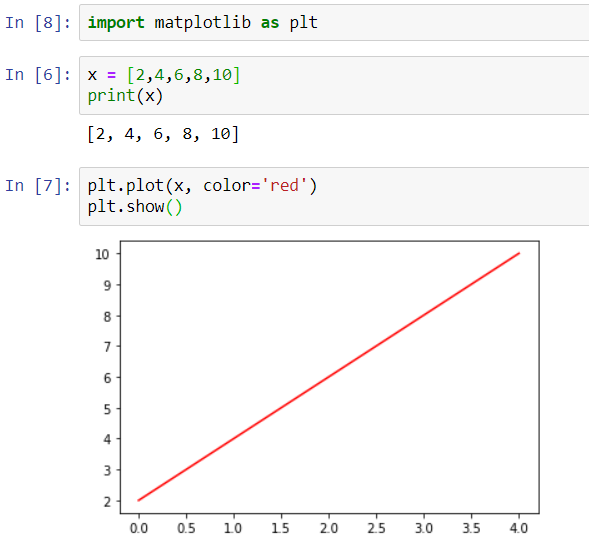Firstly we imported the library as plt and in the second step we made an integer list and as shown above, lastly, we plotted the variable ‘x’ with the help of plt.plot and visualized it using plt.show().

Now let us take a multivariable example and plot it using matplotlib:-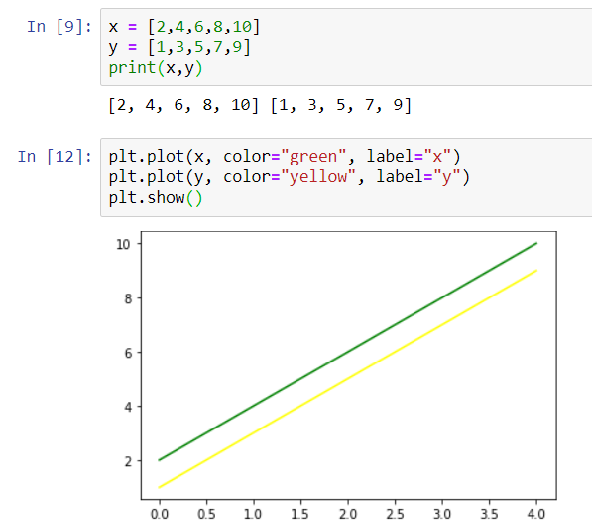Here we used two variables ‘x’ and ‘y’ and plotted them using plt.plot() with two different colors and explored it using plt.show().

There are other methods for data exploration through matplotlib.

1. ## Bar Graph Using Matplotlib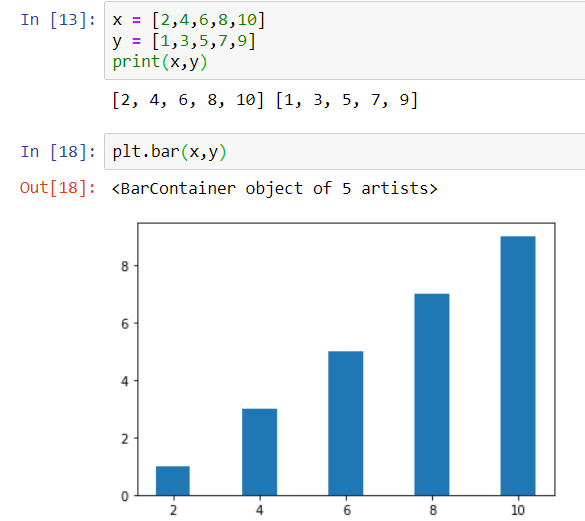1. ## Histogram Using Matplotlib Library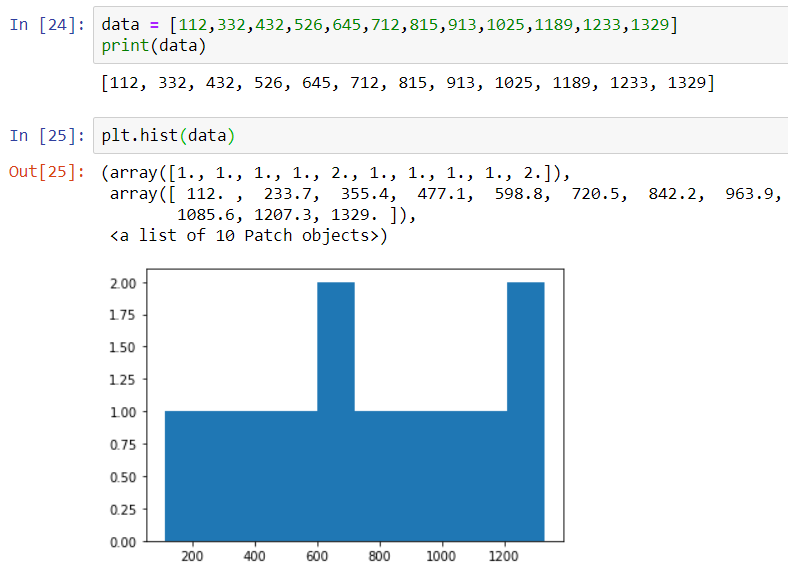1. ## Pie-chart Using Matplolib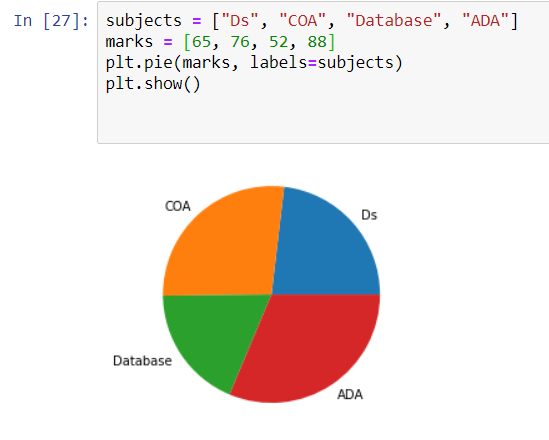## 4. Sub-plotting Using Matplotlib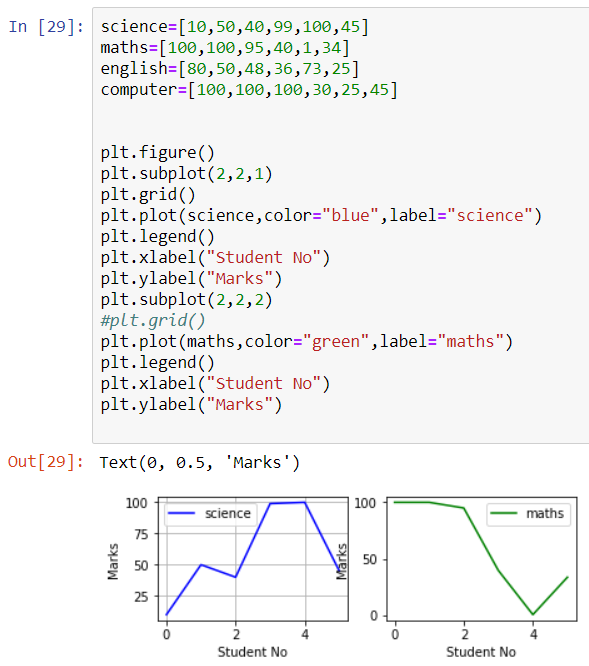I hope this blog is helpful to you, for more blogs on machine learning and Deep Learning, stay connected with us.### tanesh

Founder Of Aipoint, A very creative machine learning researcher that loves playing with the data.